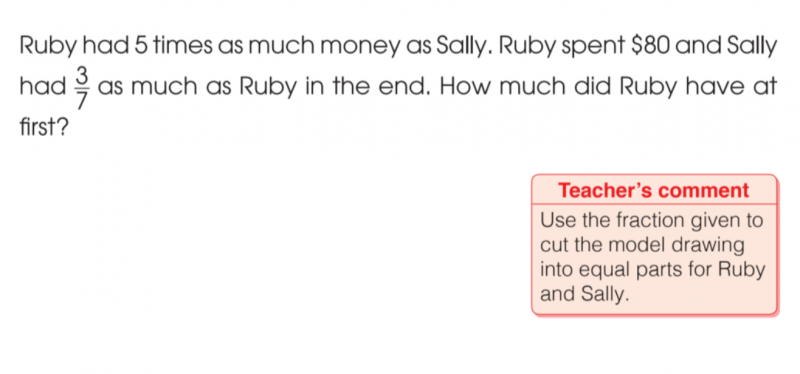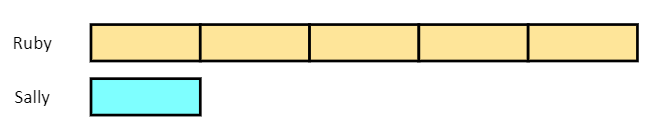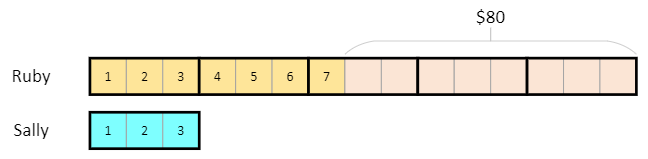# QuestionHelp with bar model

Let 3u be the amount sally has
Amount ruby has originally = 3u x 5 = 15u
Ruby’s new amount = 7u (sally had 3/7 of ruby’s new amount)
15u-80 = 7u
8u = 80
u = 10
Ruby had 15u = 15×10 = \$150

0 Replies 1 Like

Ruby    [             ][             ][             ][             ][             ]        x 5  = 15u

Sally     [             ]                                                                     x 3  = 3u

Sally remains the same but fraction has changed to 3/7

Ruby    [             ][             ][       ]                                             = 7u

Sally     [  ][  ][  ]                                                                        = 3u

Spent = 80

15u7u = 8u = 80  => u =10

Hence at first Ruby has 15u = 15 x 10 = \$150

0 Replies 1 Like
```Ruby had 5 times as much money as Sally. So we start with this:Sally had 3/7 as much as Ruby in the end.
So we modified the diagram by cutting each block
into 3 smaller pieces.Now Sally had 3 pieces and Ruby had 3 x 5 = 15 pieces.
Enumerate the 3 pieces for Sally and 7 pieces for Ruby.
Remaining 15 - 7 = 8 pieces is the \$80 spent.
So each piece is \$10.